# SSAT Upper Level Math : How to find if right triangles are congruent

## Example Questions

### Example Question #42 : Right Triangles

Given: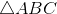, where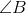is a right angle;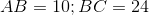;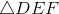, where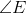is a right angle and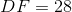;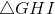, where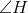is a right angle andhas perimeter 60;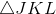, where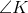is a right angle andhas area 120;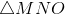, where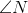is a right triangle and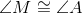Which of the following must be a false statement?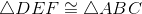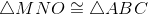All of the statements given in the other responses are possible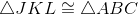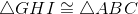Explanation:has as its leg lengths 10 and 24, so the length of its hypotenuse,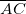, is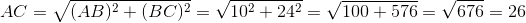Its perimeter is the sum of its sidelengths: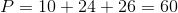Its area is half the product of the lengths of its legs: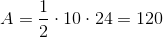andhave the same perimeter and area, respectively, as; also, betweenand, corresponding angles are congruent. In the absence of other information, none of these three triangles can be eliminated as being congruent to.

However,and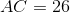. Therefore,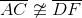. Since a pair of corresponding sides is noncongruent, it follows that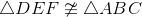.

### Example Question #51 : Right Triangles

Given:andwith right anglesand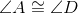.

Which of the following statements alone, along with this given information, would prove that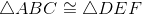?

I)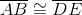II)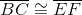III)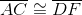Any of I, II, or III

II or III only

I or III only

I or II only

III only

Any of I, II, or III

Explanation: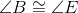since both are right angles.

Given that two pairs of corresponding angles are congruent and any one side of corresponding sides is congruent, it follows that the triangles are congruent. In the case of Statement I, the included sides are congruent, so by the Angle-Side-Angle Congruence Postulate,. In the case of the other two statements, a pair of nonincluded sides are congruent, so by the Angle-Angle-Side Congruence Theorem,. Therefore, the correct choice is I, II, or III.

### Example Question #52 : Right Triangles, whereis a right angle,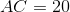, and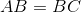.

Which of the following is true?

None of the statements given in the other choices is true.has area 100has perimeter 40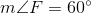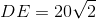has area 100

Explanation:, and corresponding parts of congruent triangles are congruent.

Sinceis a right angle, so is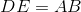and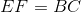; since, it follows that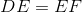is an isosceles right triangle; consequently,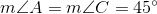.is a 45-45-90 triangle with hypotenuse of length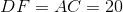. By the 45-45-90 Triangle Theorem, the length of each leg is equal to that of the hypotenuse divided by; therefore,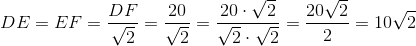is eliminated as the correct choice.

Also, the perimeter ofis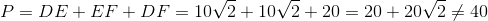.

This eliminates the perimeter ofbeing 40 as the correct choice.

Also,is eliminated as the correct choice, since the triangle is 45-45-90.

The area ofis half the product of the lengths of its legs: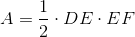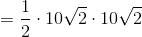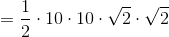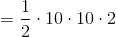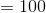The correct choice is the statement thathas area 100.